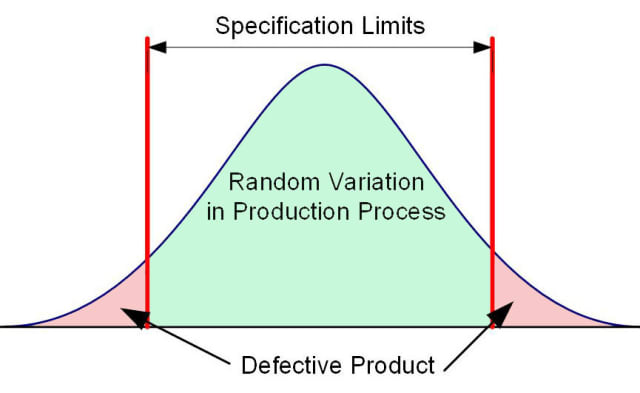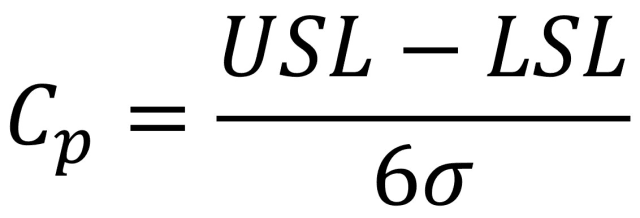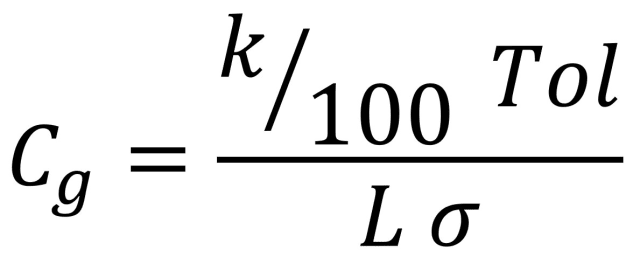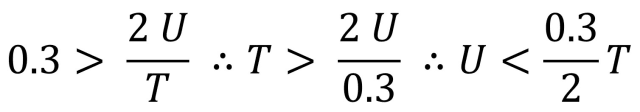Capability of Production Processes and Measurements
Dr Jody Muelaner posted on July 10, 2019 |
Ensuring accuracy is appropriate for the intended purpose.When the random variation in the production process is greater than the product’s specification limits, a defective product will be produced; the process is not capable.

In a general sense, capability is the ability to do something. Within manufacturing, capability is given a much more specific definition. It is an expression of the accuracy of a process or equipment, in proportion to the required accuracy. This can be applied to production processes, in which case any random variation and bias in the process must be significantly smaller than the product tolerance. It can also be applied to measurements, where any uncertainties in the measurement must be significantly smaller than the product tolerance or process variation that is being measured.

Capability may be expressed as a capability index; the ratio of the required accuracy to the actual accuracy. When expressed in this way, a bigger value means a more capable process. Capability may also be expressed as a capability ratio; the ratio of the actual accuracy to the required accuracy. Expressed in this way, smaller values are better, and the capability might be expressed as a percentage of the tolerance consumed by the accuracy. In both cases, additional factors are often introduced so that the index is some multiple of the raw ratio.

The level of capability that is deemed to be sufficient is different in different industrial standards. In reality, there is a trade-off between the increased cost of improving capability and the costs involved with rework, scrap and defects reaching the customer. Making an optimal trade-off therefore depends on other parameters and not simply the capability. However, for simplicity, acceptable levels for capability are often used as a major decision making tool within manufacturing.

### Process Capability

The capability of a production process to produce parts that are within tolerance is normally expressed as a capability index, involving the ratio between the part tolerance and the process variation. The part tolerance may be represented as the total range between the upper specification limit and the lower specification limit (USLLSL). Tolerance may also be combined with bias in the process so that the lower tolerance is given by the difference between the process mean (µ) and the lower specification limit (µ-LSL). Similarly, the upper tolerance is given by USL-µ.

The two most common process capability indices are Cp, sometimes referred to as the potential capability, and Cpk, which considers bias and so can be considered the actual capability. The process variation is normally represented as the standard deviation (σ). In both cases, the process variation is multiplied by a factor, such that a process capability of 1 would correspond to plus or minus three standard deviations falling within the tolerance.

The equation for Cp is:It is clear that Cp is simply the ratio of the total tolerance to six standard deviations in the process. A Cp of 1 therefore means that plus or minus 3 standard deviations of the process would fit within the tolerance limits, assuming the process is centered about the nominal value. A Cp of one is typically regarded as a bare minimum value for capability. Cp of 2, means that plus or minus 6 standard deviations of the process would fit within the tolerance limits, this is often the ultimate goal in quality engineering and is where the name for the six-sigma methodology comes from.

Cp ignores bias. This means that a process could have very little variation but consistently produce parts that are either too big or too small.

The equation for Cpk is:By considering the available tolerance as the difference between the specification limits and the process mean (µ), Cpk takes into account bias in the process. Therefore, if the Cpk is acceptable we can be fairly confident that most products will be within tolerance.

Both Cp and Cpk assume that the process output is approximately normally distributed and that the process is in control. In other words, the process should behave in a consistently random way. If a fault has developed, or there is some other significant non-random effect, that is causing the process to behave in a way that is not random, then these calculations will not be valid. Note that strictly speaking, µ should be used for the population mean and x-bar for the sample mean. However, these are often used interchangeably within capability indices.

Multiplying Cp or Cpk by three gives the sigma level (z). This is a way to remove the essentially arbitrary factors added into the equations and get back to something with a more fundamental statistical meaning. Personally, unless I have to, I don’t bother with Cp or Cpk; I’d rather just work with sigma levels. From the sigma level, it is possible to calculate the number of defective parts that can be expected, using the cumulative distribution function (CDF) for the normal distribution. For example, this can be calculated in Excel using the following formula:

=2 - 2*NORM.S.DIST(Z,TRUE)

A sigma level of 2 (z=2) would, therefore, result in a defect rate of 4.5 percent for a perfectly centred process. Dividing by three gives Cp=0.66.

A Cpk of 1.4 equates to a sigma level of 4.2 and a defect rate of less than 0.0027%. If there is significant bias then only one tail of the distribution will causing a significant chance of defects and so the defect rate may be only half this value.

### Gage Capability

Another form of capability, commonly used in manufacturing, is gage capability. This might be used to decide whether a measurement is capable of determining whether or not a part is within specification. Alternatively, it might be used to decide whether a measurement has sufficient accuracy to provide meaningful information about process variation. These are distinctly different requirements, and it is important to consider the purpose of a measurement before considering whether it is capable.

When using a measurement for product verification, we are concerned with the ratio between the product tolerance and the measurement uncertainty. When the measurement is used to monitor process variation, it is the ratio of the process variation to the measurement uncertainty that must be considered.

#### Gage Capability for Product Verification

Determining capability for product verification is somewhat easier to understand and will therefore be considered first. If the measurement variation is larger than the product tolerance it is very clear that the gage is not capable. If this is the case then even if every product is produced to exactly the nominal dimensions, the measurement results would show the parts as being randomly in or out of tolerance. At the same time, products which are well outside of tolerance could be shown to be perfect.

At the other extreme, a measurement system with no bias or random variation would provide 100 percent confidence in every measurement result’s ability to distinguish between conforming and non-conforming products.

In reality, measurement systems will usually fall somewhere between these extremes. Gage capability can provide a way to assess what is good enough. The most fundamental way to express this is as a simple capability ratio between the accuracy of the measurement system and the product’s tolerance. Typically, a ratio of 10 percent to 20 percent is regarded as acceptable. Alternatively, using the ratio between the precision and the tolerance, gives the potential capability assuming corrections are made for bias.

It is also common to use a gage capability index, which involves the ratio between the tolerance and the accuracy, together with some additional factors. The most common gage capability indices are Cg and Cgk, as defined in the Automotive Industry Action Group (AIAG) MSA Manual. Cg involves the ratio between the measurement’s precision and the product tolerance and is therefore the potential capability ignoring bias. Cgk involves the ratio between the measurement’s accuracy and the product tolerance, this is the actual capability. As with Cp and Cpk, the gage capability indices introduce some essentially arbitrary factors which can prevent understanding and subsequent statistical analysis. For example, Cg is given byThe essential ratio in this equation is given by the product tolerance (Tol) and the measurement precision (σ). If no additional factors were present then this ratio would be a sigma level (Z) which would enable further analysis to be carried out easily. However, a number of additional factors are added that have essentially arbitrary values. K is the percentage of the tolerance that is regarded as acceptable, typically 20 percent. This is divided by 100 to convert K from a percentage to a proportion. L is the number of standard deviations thought to represent the actual or target process spread, typically a value of 6 is used.

The more recent VDA-5 standard takes a somewhat different approach to measurement capability. It is also concerned with the capability of measurements for product verification. However, it makes a distinction between the measurement instrument and the measurement process. It recommends that a number of evaluations are carried out in sequence to decide whether measurements are capable of proving that the product conforms to the specification. It starts by evaluating the instrument and then moves on to consider the process. The first step is to check that the instrument’s resolution is less than 5 percent of the parts tolerance. The uncertainty of the measurement instrument’s calibration should then be evaluated using a capability ratio. If the instrument is capable, then an uncertainty evaluation should be carried out for the measurement process and this evaluated using a capability ratio. Finally, regular checks should ensure the measurement process is stable

The capability ratios (Q) for both the instrument calibration and the measurement process are the ratios between the expanded uncertainty of the instrument or process (U) and the product tolerance (T). The tolerance is expressed as a total range (T = USL-LSL) while uncertainty of measurement is a plus or minus value, therefore the uncertainty is multiplied by two to provide a consistent ratio. The expanded uncertainty is given at 95 percent confidence which means that the standard uncertainty is multiplied by two. This is done before substituting the value into this equation and shouldn’t be confused with the factor of two. The fact that an expanded uncertainty is used is the only arbitrary factor added into this equation. The ratio is multiplied by 100 to give a percentage:VDA-5 recommends that the capability ratio for the measurement instrument (QMS) is less than 15 percent and for the measurement process (QMP) it is less than 30 percent.

Using these recommended limits, and rearranging the equation, it is also possible to express the capability of a measuring system or process as the minimum tolerance or maximum uncertainty. For example, for a measurement process:#### Gage Capability for Process Monitoring

A measurement used to monitor the variation in a production process should have considerably less variation than the process being monitored. This is essentially about signal to noise. If random variations in the measurement system are similar in size to the random variations in the process, then it is very difficult to observe what the process is doing.

This type of gage capability is typically monitored using a Gage R&R study. This involves measuring a number of parts, typically 10, representing the normal variation of the production process. Each part is measured a number of times by a number of operators. Analysis of Variance (ANOVA) is then used to separate out actual part-to-part variation from measurement variation.

AIAG guidelines state that measurement variation (Total Gage R&R standard deviation) should ideally be less than 10 percent of the study variation. Up to 30 percent may still be acceptable if it cannot be easily improved. A major weakness with this approach is that it depends on a sample of 10 parts to determine the process variation.

### Beyond capability – optimized processes

Achieving acceptable levels for capability indices, according to particular standards, does not infer that optimal selections of instruments and machines have been made. In practice, industrial engineers are frequently required to select from several instruments or machines, all of which may either be capable or may not be capable. This involves subjective judgements about what is ‘good enough’ and what level of expenditure is justified. It is, however, possible to make optimal trade-offs between cost and quality. This can be achieved by using sigma levels and Bayesian statistics to calculate the probability of false decisions. Costs associated with scrap and defects reaching the customer can then be used to calculate a quality adjusted cost per unit of product sold. Optimal instrument and machine selections, as well as product acceptance decisions, can then be made so as to minimize this cost. I’ll look at this state of the art approach to quality in more detail in a future post.

 Recommended For You